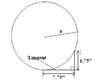# Radius of a circle that intersects two points on a right triangle.

dgoldman86
I'm trying to figure out the radius of a circle that intersects two points on a right triangle. One side of the triangle is tangent to the circle and the other intersects it. I have attached an image that helps further explain what I'm talking about. Knowing what I have listed in the image is there anyway to determine the radius of that circle?

Thanks.

#### Attachments

•Circle.JPG
7.4 KB · Views: 416

Call the vertex of your right triangle $(c_1,r,c2)$ with $c_1$ tangent to the circle and $r$ the vertex at the right angle. Let $o$ be the center of your circle.
Now construct the square $(o,c_1,r,s)$.
Observe that $c_2$ is on the square side $(r,q)$ and use pythagoras.